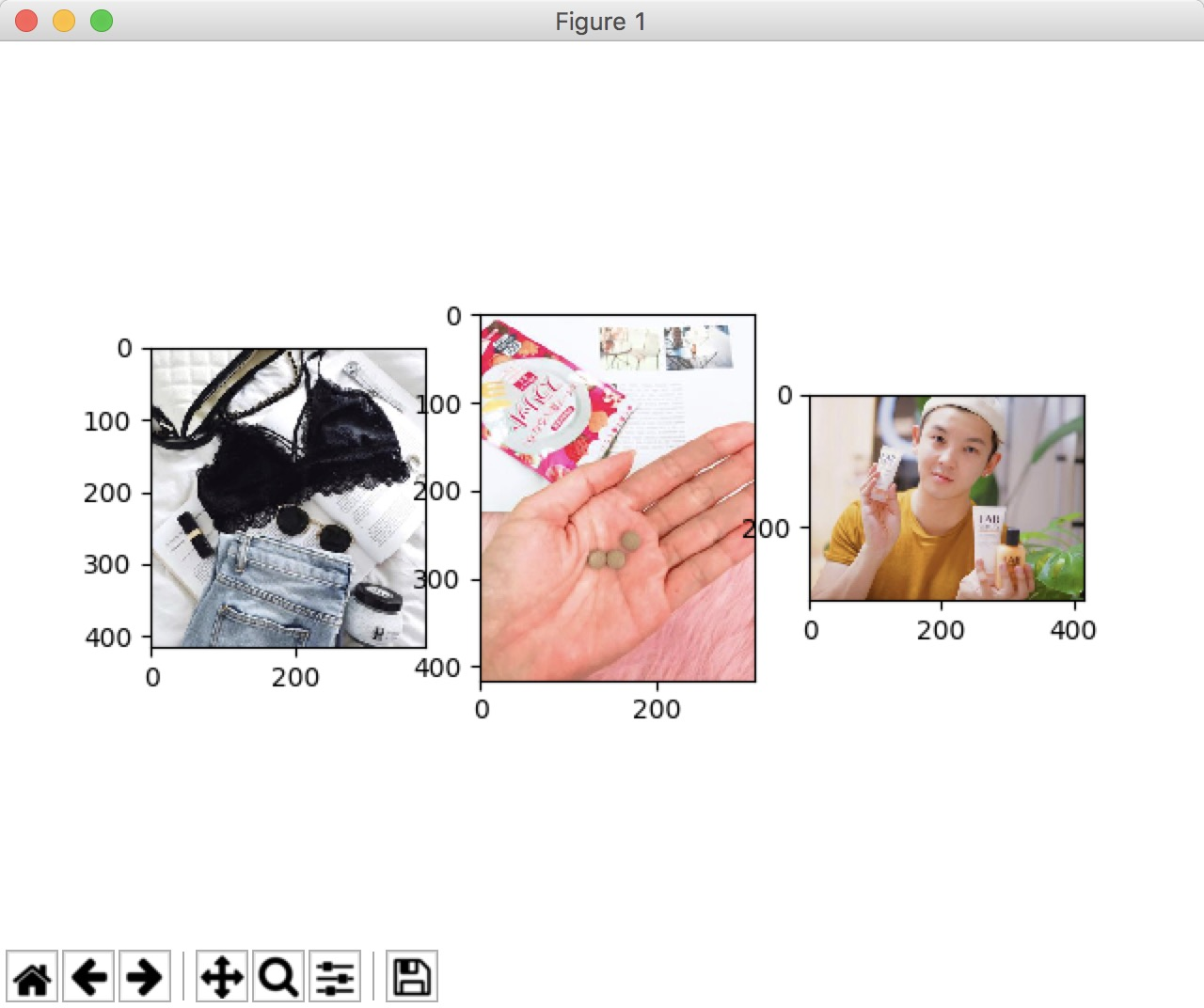• 加载的数据集中已自动生成X张图片 import matplotlib.pyplot as plt from scipy.io import loadmat mat = loadmat('data1.mat') # 假设文件中有32张图片 img= mat['img'] plt.figure() for i in range(1,X): # X为...
*1.加载的数据集中已自动生成X张图片
import matplotlib.pyplot as plt

img= mat['img']
plt.figure()
for i in range(1,X):  # X为图片的个数
plt.subplot(a,b,i-1)  # a,b 分别为图片集的行数，列数
plt.imshow(img[i-1])
plt.show()

这就是显示的结果:

在代码中加入下面两行则没有坐标了

for i in range(1,X):  # X为图片的个数
plt.subplot(a,b,i-1)  # a,b 分别为图片集的行数，列数
plt.imshow(img[i-1])
plt.xticks([])
plt.yticks([])
plt.show()


展开全文python
• 在网上查找了好多用Matplotlib显示多张图像到一张图像上的方法,总是报各种错误,在这里,将最笨拙的方法贴出来,供各位网友参考,欢迎大家在评论区推荐更简洁的方法… import os import numpy as np from PIL import ...
在网上查找了好多用Matplotlib显示多张图像到一张图像上的方法,总是报各种错误,在这里,将最笨拙的方法贴出来,供各位网友参考,欢迎大家在评论区推荐更简洁的方法…
import os
import numpy as np
from PIL import Image
import matplotlib.pyplot as plt
import cv2

plt.figure()

# print(img.shape)
b,g,r = cv2.split(img)
img1 = cv2.merge([r,g,b])
plt.subplot(2,3,1)
plt.title(str(1))
plt.axis('off')
plt.imshow(img1)

# img= Image.open(img_p)
# print(img.shape)
b,g,r = cv2.split(img)
img1 = cv2.merge([r,g,b])
plt.subplot(2,3,2)
plt.title(str(2))
plt.axis('off')
plt.imshow(img1)

# img= Image.open(img_p)
# print(img.shape)
b,g,r = cv2.split(img)
img1 = cv2.merge([r,g,b])
plt.subplot(2,3,3)
plt.title(str(3))
plt.axis('off')
plt.imshow(img1)

b,g,r = cv2.split(img)
img1 = cv2.merge([r,g,b])
plt.subplot(2,3,4)
plt.title(str(4))
plt.axis('off')
plt.imshow(img1)

b,g,r = cv2.split(img)
img1 = cv2.merge([r,g,b])
plt.subplot(2,3,5)
plt.title(str(5))
plt.axis('off')
plt.imshow(img1)

b,g,r = cv2.split(img)
img1 = cv2.merge([r,g,b])
plt.subplot(2,3,6)
plt.title(str(6))
plt.axis('off')
plt.imshow(img1)

plt.tight_layout()  # 调整整体空白
wspace=None, hspace=None)  # 调整子图间距
'''
# 参数说明：
top、bottom、left、right：整个图距离上下左右边框的距离
wspace、hspace：这个才是调整各个子图之间的间距
wspace：调整子图之间的横向间距
hspace：调整子图之间纵向间距

'''
plt.show()

展开全文• 问题： 使用matplotlib的pyplot.savefig保存图片时，前面的图会不断叠加到后面的图中。 原因： savefig方法保存图片并不会重置画布，所以导致图片的相互叠加。 解决方法： 保存图片后，使用plt.clf()重置画布。 ...
问题： 使用matplotlib的pyplot.savefig保存图片时，前面的图会不断叠加到后面的图中。 原因： savefig方法保存图片并不会重置画布，所以导致图片的相互叠加。 解决方法： 保存图片后，使用plt.clf()重置画布。
展开全文• 图合一(subplot)2.分格显示2.1.subplot2grid方法2.2.gridspec方法2.3.subplots方法3.图中有图(plot in plot)4.设置双坐标轴 1.图合一(subplot) matplotlib 是可以组合许多的小图, 放在一张大图里面显示的. 使用...
• import matplotlib matplotlib.use('TkAgg') import matplotlib.pyplot as plt subplot，131，表示1行3列的第1个；其余类似。 plt.subplot(131) plt.imshow(data.asnumpy()) plt.subplot(132) plt.imshow(....
Mac中，使用TkAgg样式
import matplotlib
matplotlib.use('TkAgg')

import matplotlib.pyplot as plt
subplot，131，表示1行3列的第1个；其余类似。
plt.subplot(131)
plt.imshow(data.asnumpy())
plt.subplot(132)
plt.imshow(data.asnumpy())
plt.subplot(133)
plt.imshow(data.asnumpy())
输出：展开全文subplot
• CIFAR10读进来的图像的的维度是（50000,32,32,3），插入以下代码就可以用了， ...选取前32显示 plt.figure() for i in range(1,32): plt.subplot(4,8,i) plt.imshow(img_test[i-1]) plt.show() 这...
• Matplotlib在一张画布上画个图的两种方法，plt.subplot,plt.subplots。 目录回顾plt.subplots()画法plt.subplot()画法保存 回顾 之前也用过plt.subplots()在一张图上画过个图，今天看到用plt.subplot()的画法想...
• 其实，利用python 的matplotlib包下的subplot函数可以将个子图放在同一个画板上。在此之前，我们先来看一个案例： import matplotlib.pyplot as plt import numpy as np plt.rcParams[‘font.sans-seripython 数据分析
• 我现在有一组图片，一共100张图片，每张大小是200*200，即imgs.shape=100*200*200*3 (注意通道数在最后一维)。 我需要同时绘制这100张图片，而且每张图片需要写上对应的名字，所以这里假设你已经准备好了你的图像...
• 做数据分析，还有机器学习的收敛性，准确性分析时，往往需要将一些数据图形化，以曲线的形式显示出来，下面就介绍两种方式来实现这个小问题，一种...import matplotlib.pyplot as plt x = np.linspace(0,2,100) fi...
• python matplotlib在一张画布上画个图 - fig.add_subplot() import matplotlib.pyplot as plt fig = plt.figure(figsize=(10, 10),dpi=100) # 图一 ax1 = fig.add_subplot(2, 2, 1) #2行 2列 索引为1 ax1.imshow...数据可视化
• 7张图，每行两，最后一张位于中间 方法 使用matplotlib.gridspec 分成四行四列 gs = gridspec.GridSpec(4, 4) 每行第一张图占0,1 位；第二张图占2,3位；最后一行的图占1,2位 即：plt.subplot(gs[行, 列]) # ...
• python使用opencv或matplotlib多张图片显示在一个窗口内的方法 比如你某个文件夹下有多张图片，你不想imshow一张一张的显示，你想放在一个窗口中显示。 或者是把多张图片放在一个窗体内对比展示，而不是同时打开...
• 3、使用Matplotlib展示一张图片     1、cv2展示一张图片 代码如下： import cv2 as cv import numpy as np from matplotlib import pyplot as plt path="D:\\openCV\\opencv\\sources\\samples\\data\...
• 在这篇文章中介绍了如何生成张图，但是很时候我们需要将图片综合对比展示，因此需要合并在一张图里。 以下给了2种方法：法一是通常展示多行列的思路，参考文1；法二可以用来发单行/单列的长图，参考文2。 ...python
• 文章目录一、在pytorch中紧凑画出子图（1）在一行里画出张图像和对应标签1）代码2）效果展示色偏原因分析：（2）以矩阵的形式展示多张图片1）代码2）效果展示二、在matplotlib中紧凑画出子图（1）区分 subplot 和 ...python pytorch 深度学习
• 1. 保存单张图片 保存单张图片代码如下： # acc是我跑的神经网络的历代准确率 ...2. 保存多张图片 跑神经网络的时候，我需要保存损失函数下降曲线和准确率上升曲线，如果按1中的代码，两者直接堆叠一起神经网络 深度学习 python
• 2. 图片生成代码介绍 1. 导入库介绍 import os import inspect import pandas as pd import numpy as np from datetime import datetime # 在jupyter notebook中显示图像 import matplotlib.pyplot as plt %...python 数据分析 可视化
• matplotlib 显示两张图片，折线图 和 scipy 显示两张图片的代码： import numpy as np from scipy.misc import imread, imsave, imresize import matplotlib.pyplot as plt img = imread('cat.jpg') print(img....
• 使用matplotlib的pyplot.savefig保存图片时，前面的图会不断叠加到后面的图中。 原因： savefig方法保存图片并不会重置画布，所以导致图片的相互叠加。 解决方法： 保存图片后，使用plt.clf()重置画布。 转载于...
• 比如你某个文件夹下有多张图片，你不想imshow一张一张的显示，你想放在一个窗口中显示。 或者是把多张图片放在一个窗体内对比展示，而不是同时打开个窗体。 如果用单纯的用cv2.imshow打开个窗体来显示多张图片是...python
• """ 一个窗口中绘制多张图像: Args: titles: 图像对应标题、 num_cols: 每行最多显示多少图像 scale: 用于调整图窗大小，测试时2.5会比较好 """ num_imgs = len(images) if num_cols is None: numpython
• import matplotlib.pyplot as plt import numpy as np import math plt.rcParams['font.sans-serif']=['SimHei'] # 用来正常显示中文标签 plt.rcParams['axes.unicode_minus']=False # 用来正常显示负号 x = np....
• 1. 代码 import matplotlib.pyplot as plt # 例子 1: ############################### ...# plt.subplot(行数，列数，第几张)两行两列一共4张图片 plt.subplot(2, 2, 1) plt.plot([0, 1], [0, 1]) plt.subplo...
• with PdfPages(path + "\\data\\" + "mult.pdf") as pdf: 这行代码的意思就是循环把图片插入到指定路径的pdf中， 注意：pdf.savefig()一定要写这行代码，并且plt.close() 关闭lot，要不第二张图就和前一张图重合了...
• 保存 matplotlib 生成的个图，防止前后覆盖 采用 下面代码，每次保存后，对建的区域清空 plt.clf() # 添加上这一行，画完第一个图后，重置一下 例子 import matplotlib.pyplot as plt ## 图A saveName = ‘A’ ......